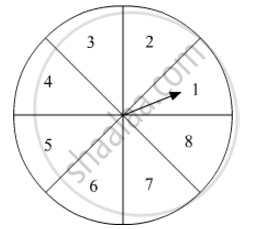# A game of chance consists of spinning an arrow on a circular board, divided into 8 equal parts, which comes to rest pointing at one of the numbers 1, 2, 3, ..., 8 (Fig. 9), which are equally likely outcomes. - Mathematics

A game of chance consists of spinning an arrow on a circular board, divided into 8 equal parts, which comes to rest pointing at one of the numbers 1, 2, 3, ..., 8 (Fig. 9), which are equally likely outcomes. What is the probability that the arrow will point at (i) an odd number (ii) a number greater than 3 (iii) a number less than 9.#### Solution

Arrow can come to rest at any of the numbers 1, 2, 3, 4, 5, 6, 7 and 8.

Total number of events = 8

(i) There are four odd numbers 1, 3, 5 and 7.
Probability that the arrow will point at an odd number is given by P (Arrow point at odd number)4/8=1/2

(ii) There are five numbers greater than 3, that is, 4, 5, 6, 7 and 8.
Probability that the arrow will point at a number greater than 3 is given by P (Arrow point at a number greater than 3) =
5/8

(iii) All the numbers are less than 9.
Probability that the arrow will point at a number less than 9 is given by P (Arrow point at a number less than 9)
8/8=1

Concept: Basic Ideas of Probability
Is there an error in this question or solution?
2015-2016 (March) Delhi Set 1
Share